## Topic 6.3: Transformations to Linear Regression (Theory)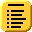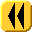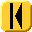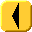Introduction Notes Theory HOWTO Examples Engineering Error Questions Matlab Maple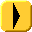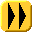# Assumptions

Suppose we have collected a number of data points which are known to follow an exponential curve of the form y = a eb x. That the data is exponential must be derived from the model or by observation.

# Derivation

Given such data, if we take the natural logarithm of both sides of the equation y = a eb x, we get log(y) = log(a eb x) = log(a) + log(eb x) = log(a) + b x.

If we represent φ = log(y) and α = log(a), then this equation φ = α + b x, which is of the appropriate form for using least squares.

Using least squares, once we find α, we may set a = eα and therefore we have the coefficients a and b which describe the exponential curve which most closely passes through the given data points (xi, yi) for i = 1, 2, ..., n.